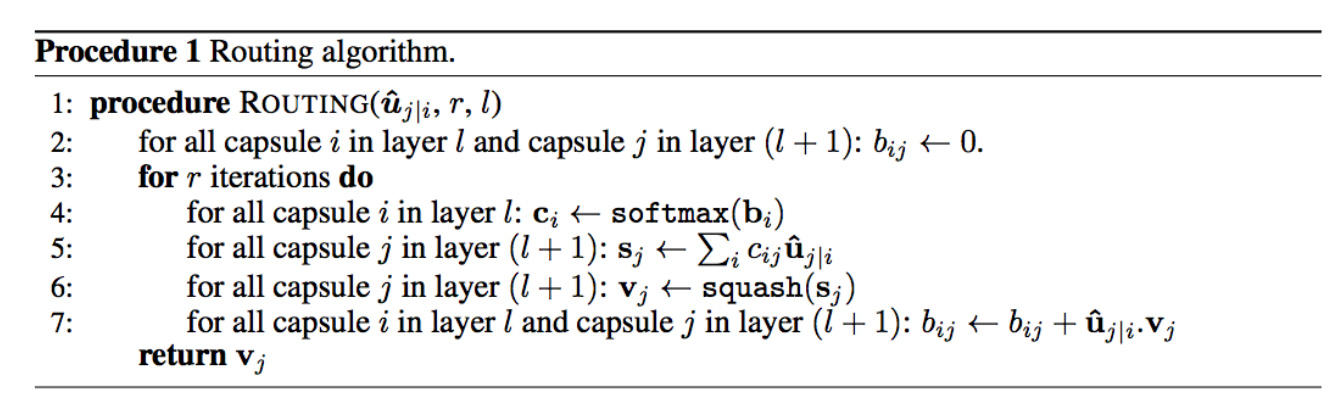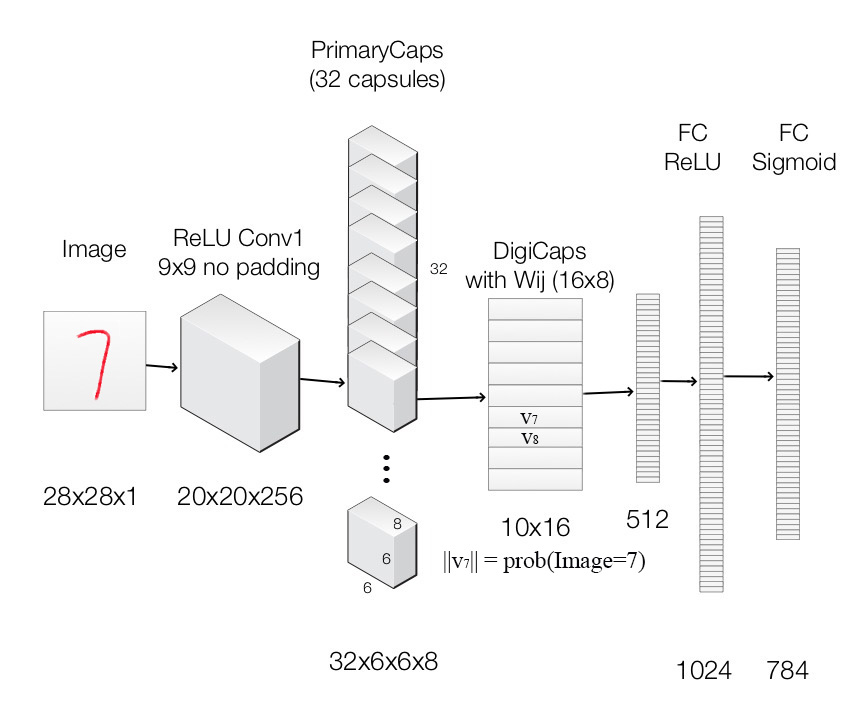# Capsule Networks给我的思考

Posted by SkyHigh on April 6, 2018

# Capsule Networks

## 动态路由（dynamic routing）

### iterative dynamic routingpaper中给出迭代次数为3次。训练和测试过程都需要做动态路由。

## 小小实验Layer Name Apply Output shape
Image Raw image array 28x28x1
ReLU Conv1 Convolution layer with 9x9 kernels output 256 channels, stride 1, no padding with ReLU 20x20x256
PrimaryCapsules Convolution capsule layer with 9x9 kernel output 32x6x6 8-D capsule, stride 2, no padding 6x6x32x8
DigiCaps Capsule output computed from a Wij (16x8 matrix) between ui and vj (i from 1 to 32x6x6 and j from 1 to 10). 10x16
FC1 Fully connected with ReLU 512
FC2 Fully connected with ReLU 1024
Output image Fully connected with sigmoid 784 (28x28)

### transformation

# (batch_size, 1, input_num_capsule, input_dim_capsule)
expand_inputs = K.expand_dims(inputs, axis=1)
# (batch_size, num_capsule, input_num_capsule, input_dim_capsule)
expand_inputs = K.tile(expand_inputs, (1, self.num_capsule, 1, 1))
# (batch_size, num_capsule, input_num_capsule, dim_capsule)
u_hat = K.map_fn(lambda x: K.batch_dot(x, self.W, axes=[2, 3]), expand_inputs)


### squash

def squash(s, axis=-1):
"""
Squash function. This could be viewed as one kind of activations.
"""
squared_s = K.sum(K.square(s), axis=axis, keepdims=True)
scale = squared_s / (1 + squared_s) / K.sqrt(squared_s + K.epsilon())
return scale * s


### dynamic routing

# (batch_size, num_capsule, input_num_capsule)
b = K.zeros((K.shape(u_hat), self.num_capsule, self.input_num_capsule))
for i in xrange(self.num_routing):
# (batch_size, num_capsule, input_num_capsule)
c = softmax(b, axis=1)
# (batch_size, num_capsule, dim_capsule)
s = K.batch_dot(c, u_hat, axes=[2, 2])
squashed_s = squash(s)
if i < self.num_routing - 1:
# (batch_size, num_capsule, input_num_capsule)
b += K.batch_dot(squashed_s, u_hat, axes=[2, 3])
return squashed_s


### margin loss

def margin_loss(y, pred):
"""
For the first part of loss(classification loss)
"""
return K.mean(K.sum(y * K.square(K.maximum(0.9 - pred, 0)) + \
0.5 *  K.square((1 - y) * K.maximum(pred - 0.1, 0)), axis=1))